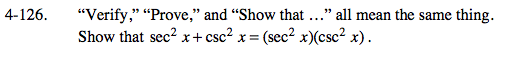### Home > PC > Chapter 4 > Lesson 4.3.1 > Problem4-126

4-126.

“Verify,” “Prove,” and “Show that …” all mean the same thing. Show that sec2 x + csc2 x = (sec2 x)(csc2 x). Homework Help ✎Change only the left side until you get the right side.

$\frac{1}{\cos^2x}+\frac{1}{\sin^2x}$

$\frac{\sin^2x+\cos^2x}{\cos^2x\sin^2x}$